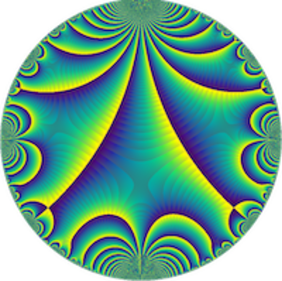# Partial classicality of Hilbert modular forms (Chi-Yun Hsu, Université de Lille)

Séminaire « Arithmétique »
M2 Kampé de Fériet
When studying modular forms, we sometimes want to regard them as in the larger space of p-adic overconvergent modular forms, so that p-adic analytic techniques can be applied. It is hence important to have the philosophy of classicality theorems: when the p-adic valuation of Up-eigenvalue is small compared to the weight (called a small slope condition), an overconvergent Up eigenform is automatically classical. In the situation of Hilbert modular forms associated with a totally real field F of degree g over Q, there is the notion of I-classical overconvergent forms, for each subset I of the g real embeddings of F. When I is the empty set, this is the usual notion of overconvergence. We establish a partial classicality theorem: when an overconvergent form satisfies a weaker small slope condition depending on I, it is automatically I-classical.